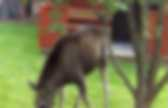# Worksheets

## Tips

Annelis matteböcker. Maths. Mental Maths Worksheets When a child practices mental maths maths becomes quicker and simpler = maths mastery!My girls look at their maths charts (mini office A5 maths) too much, so I made some fun worksheets for practice. These worksheets reinforce adding, subtracting, multiplying and dividing with just a few minutes practice every day. (Click the titles for your download.) Maths Rockets ~ for adding/ subtracting or multiplying numbers on the side to equal the number in the roof (great for bonds) Maths Butterflies ~ 5 sums for each butterfly, so good for quick reviews Maths Corners ~ 4 sums for each number, also a quick worksheet Maths In&Out ~ top row of random numbers “in” to work with a number and operation, and write the answer as “out” Maths Caterpillars ~ each caterpillar has random numbers or operations, from simple to complex Maths Amazing Squares ~ brilliant for bonds to 10 ~ all rows add to 10, up, down or sideways!

Maths Manipulatives: Maths Number Value Cards Math Bonds of 10 puzzle. Skip Counting. Skip Counting by 2 and 5 – Worksheet Skip Counting by 2, 3, 4, 5, 6 and 7 – Worksheet Skip Counting by 10, 100 and 1000 – Worksheet Skip Counting by 6, 7, 8 and 9 – Worksheet Skip Counting by 2, 3, 4 and 5 – Worksheet.Color by number coloring pages. Free Math worksheets, Free phonics worksheets, Math Games and Online activities and Quizzes. Problemlösning 2ans tabell by Susanne Jönsson. Easter Worksheets. Our Easter worksheets offer some fun and interesting activities for the classroom at Easter time - nothing too strenuous, but covering a range of skills.You might also want to look at our Easter handwriting worksheets. Kids can colour in this cute picture of a bunny - perfect for Easter or any time - but first they need to solve some simple sums to work out the right colours to use! Here's one of our fact-finding worksheets for the chick. Get the kids to do some research and then fill it in. Count the Easter pictures and write the correct number in the box.

Here's our trickiest Easter counting worksheet! Complete the Easter egg by copying one half to the other with this fun symmetry worksheet. Test the kids on their ordinal numbers with the help of this worksheet with an Easter theme. We have three variations of this Easter word worksheet so you can choose the most appropriate for your child. Complete the rabbit by copying one half to the other with this fun symmetry worksheet. Math Worksheets: Grade 3. Free Lapbooks and Free Templates, Foldables, Printables, Make Your Own Lapbook.

Free Math Worksheets, Problems and Practice. School stuff. Free Math Worksheets. Mathematics worksheets - create free maths worksheets, puzzles and games. Create Custom Pre-Algebra, Algebra 1, Algebra 2, and Geometry Worksheets. This page contains a collection of free and printable math worksheets for grade 1 students and beyond. Math worksheets.Numbers, Counting, Place Value and Ordering worksheets Counting by 1 - Number line Counting and coloring - Numbers activity Counting fruits - Basic counting and addition Comparing numbers - More than/less than Color by number - Subtraction from 100 activity Ordering numbers - Numbers up to 100 Ice-cream color activity - Comparing and adding numbers Odd and even numbers - Up to 100 Ordinal numbers - Color activity Tens and ones - Place value worksheet Addition, Subtraction and Multiplication Worksheets Basic addition chart - Numbers up to 100 Color by numbers - Subtraction witin 15 Adding 4 to numbers - Basic addition worksheet Adding 6 to numbers - Basic addition worksheet.

Learn to Read: Free Phonics worksheets, Phonics Games, Phonics Activities and Phonics Flashcards. Phonics Flashcards Activities CVC Flashcards -with uppercase alphabets CVC Flashcards -with lowercase alphabets Long vowel (magic E) flashcards -with lowercase alphabets R Controlled words flashcards Lowercase LettersUppercase LettersAlphabet Train GameAlphabet Ordering Games Kids Phonics learning games: Phonics Games To link to this page, copy the following code to your site: <a href="WorksheetWorks.com. Mathmaster.org - Create math worksheets for free. Math-drill.com. Fiches maternelles de graphisme grande section moyenne section ecriture. Dynamically Created Math Worksheets.